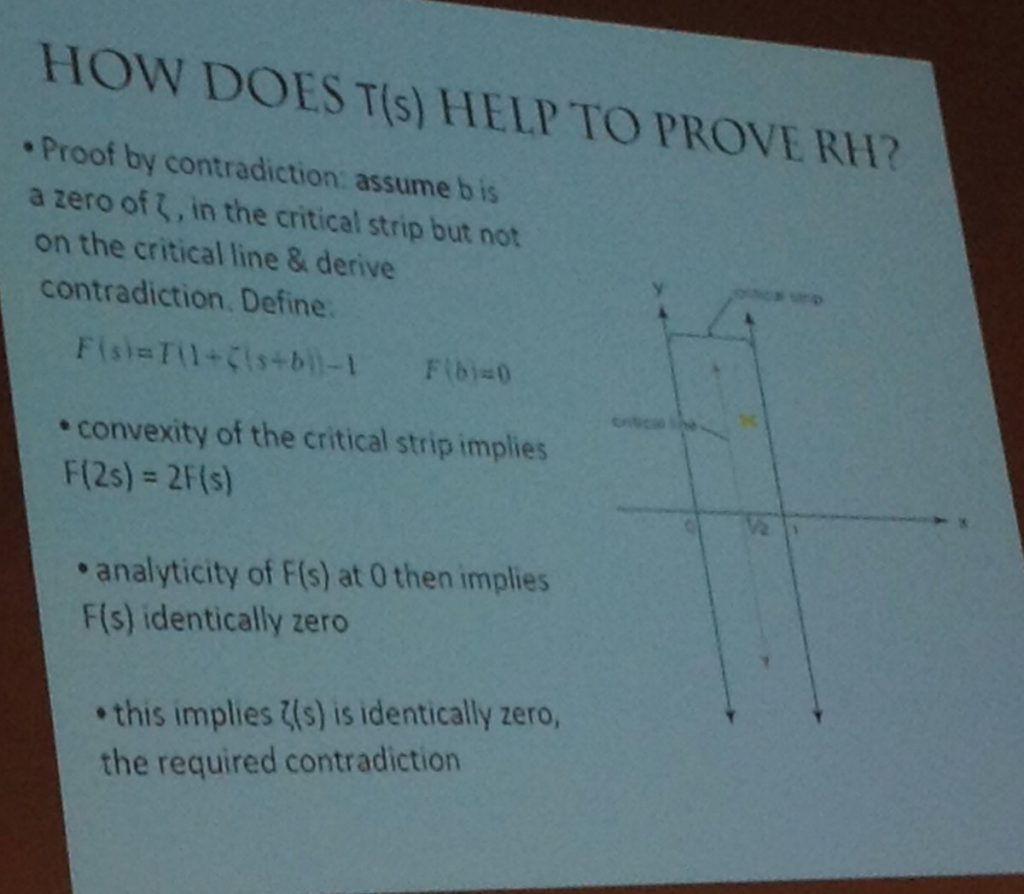Michael Atiyah, 89, is one of the greatest living mathematicians. Which is why the world pays attention when he claims to have solved what is perhaps the greatest outstanding problem in mathematics, the Riemann hypothesis.

Here is a simple sum: $$1+\frac{1}{2^2}+\frac{1}{3^2}+…$$. It is actually convergent: The result is $$\pi^2/6$$.

Other, similar sums also converge, so long as the exponent is greater than 1. In fact, we can define a function:

\begin{align*}\zeta(x)=\sum\limits_{i=1}^\infty\frac{1}{i^x}.\end{align*}

Where things get really interesting is when we extend the definition of this $$\zeta(x)$$ to the entire complex plane. As it turns out, its analytic continuation is defined almost everywhere. And, it has a few zeros, i.e., values of $$x$$ for which $$\zeta(x)=0$$.

The so-called trivial zeros of $$\zeta(x)$$ are the negative even integers: $$x=-2,-4,-6,…$$. But the function also has infinitely many nontrivial zeros, where $$x$$ is complex. And here is the thing: The real part of all known nontrivial zeros happens to be $$\frac{1}{2}$$, the first one being at $$x=\frac{1}{2}+14.1347251417347i$$. This, then, is the Riemann hypothesis: Namely that if $$x$$ is a non-trivial zero of $$\zeta(x)$$, then $$\Re(x)=\frac{1}{2}$$. This hypothesis baffled mathematicians for the past 130 years, and now Atiyah claims to have solved it, accidentally (!), in a mere five pages. Unfortunately, verifying his proof is above my pay grade, as it references other concepts that I would have to learn first. But it is understandable why the mathematical community is skeptical (to say the least).A slide from Atiyah’s talk on September 24, 2018.

What is not above my pay grade is analyzing Atiyah’s other claim: a purported mathematical definition of the fine structure constant $$\alpha$$. The modern definition of $$\alpha$$ relates this number to the electron charge $$e$$: $$\alpha=e^2/4\pi\epsilon_0\hbar c$$, where $$\epsilon_0$$ is the electric permeability of the vacuum, $$\hbar$$ is the reduced Planck constant and $$c$$ is the speed of light. Back in the days of Arthur Eddington, it seemed that $$\alpha\sim 1/136$$, which led Eddington himself onto a futile quest of numerology, trying to concoct a reason why $$136$$ is a special number. Today, we know the value of $$\alpha$$ a little better: $$\alpha^{-1}\simeq 137.0359992$$.

Atiyah produced a long and somewhat rambling paper that fundamentally boils down to two equations. First, he defines a new mathematical constant, denoted by the Cyrillic letter $$\unicode{x427}$$ (Che), which is related to the fine structure constant by the equation

\begin{align*}\alpha^{-1}=\frac{\pi\unicode{x427}}{\gamma},\tag{1.1*}\end{align*}

where $$\gamma=0.577…$$ is the Euler–Mascheroni constant. Second, he offers a definition for $$\unicode{x427}$$:

\begin{align*}\unicode{x427}=\frac{1}{2}\sum\limits_{j=1}^\infty 2^{-j}\left(1-\int_{1/j}^j\log_2 x~dx\right).\tag{7.1*}\end{align*}

(The equation numbers are Atiyah’s; I used a star to signify that I slightly simplified them.)

Atiyah claims that this sum is difficult to calculate and then goes into a long-winded and not very well explained derivation. But the sum is not difficult to calculate. In fact, I can calculate it with ease as the definite integral under the summation sign is trivial:

\begin{align*}\int_{1/j}^j\log_2 x~dx=\frac{(j^2+1)\log j-j^2+1}{j\log 2}.\end{align*}

After this, the sum rapidly converges, as this little bit of Maxima code demonstrates (NB: for $$j=1$$ the integral is trivial as the integration limits collapse):

(%i1) assume(j>1);
(%o1)                               [j > 1]
(%i2) S:1/2*2^(-j)*(1-integrate(log(x)/log(2),x,1/j,j));
log(j) + 1
---------- + j log(j) - j
(- j) - 1          j
(%o2)             2          (1 - -------------------------)
log(2)
(%i3) float(sum(S,j,1,50));
(%o3)                         0.02944508691740671
(%i4) float(sum(S,j,1,100));
(%o4)                         0.02944508691730876
(%i5) float(sum(S,j,1,150));
(%o5)                         0.02944508691730876
(%i6) float(sum(S,j,1,100)*%pi/%gamma);
(%o6)                         0.1602598029967022


Unfortunately, this does not look like $$\alpha^{-1}=137.0359992$$ at all. Not even remotely.

So we are all left to guess, sadly, what Atiyah was thinking when he offered this proposal.

We must also remember that $$\alpha$$ is a so-called “running” constant, as its value depends on the energy of the interaction, though presumably, the constant in question here is $$\alpha$$ in the infrared limit, i.e., at zero energy.